## research papersJOURNAL OFSYNCHROTRONRADIATION
ISSN: 1600-5775
Volume 26| Part 3| May 2019| Pages 729-736

## A piezoelectric deformable X-ray mirror for phase compensation based on global optimization

aShanghai Synchrotron Radiation Facility, Shanghai Advanced Research Institute, Chinese Academy of Sciences, Zhangheng Road 239, Pudong District, Shanghai 201204, People's Republic of China, and bShanghai Institute of Applied Physics, Chinese Academy of Sciences, Jiading District, Shanghai People's Republic of China
*Correspondence e-mail: jianghui@sinap.ac.cn

(Received 30 November 2018; accepted 27 February 2019; online 9 April 2019)

As a strong tool for the study of nanoscience, the synchrotron hard X-ray nanoprobe technique enables researchers to investigate complex samples with many advantages, such as in situ setup, high sensitivity and the integration of various experimental methods. In recent years, an important goal has been to push the focusing spot size to the diffraction limit of ∼10 nm. The multilayer-based Kirkpatrick–Baez (KB) mirror system is one of the most important methods used to achieve this goal. This method was chosen by the nanoprobe beamline of the Phase-II project at the Shanghai Synchrotron Radiation Facility. To overcome the limitations of current polishing technologies, the use of an additional phase compensator was necessary to decrease the wavefront distortions. In this experiment, a prototype phase compensator has been created to show how to obtain precise wavefront compensation. With the use of finite-element analysis and Fizeau interferometer measurements, some important factors such as the piezoresponse, different actuator distributions, stability and hysteresis were investigated. A global optimization method based on the measured piezoresponse has also been developed. This method overcame the limitations of the previous local algorithm related to the adjustment of every single actuator for compact piezoelectric layouts. The mirror figure can approach a target figure after several iterations. The figure difference can be reduced to several nanometres, which is far better than the mirror figure errors. The prototype was also used to successfully compensate for the real wavefront errors from upstream and for its own figure errors, measured using the speckle scanning technique. The residual figure error was reduced to a root-mean-square value of 0.7 nm.

### 1. Introduction

Nanoscience has been recognized as one of the future main driving forces of the world's economic development in the next few decades. As is commonly known, when a material is small enough at the nanoscale it has many new properties that are completely different from the properties of the macroscopic objects. To meet the needs of nanoscale research, nanometre-resolution microscopy has been widely developed. Compared with a high-resolution electron probe, hard X-rays have the advantages of high energy, strong penetration, high focal depth and non-destructiveness. Hard X-ray focusing can further promote spatial resolution, spectroscopy and diffraction measurement techniques (Ice et al., 2011). In the past several decades, third-generation synchrotron radiation sources and free-electron lasers have been developing rapidly. With the brightness, coherence and stability of a light source, the value of hard X-ray focusing is fully embodied. At present, most of the world's light sources give priority to the design and construction of micrometre, sub-micrometre and even nano-focusing beamlines. There are many ways to achieve sub-micrometre focusing, such as the mechanical bending of Kirkpatrick–Baez (KB) mirrors, compound refractive lenses and capillary optics. The focus of these components is mostly satisfied by the geometric compression ratio in the beamline design. In recent years, researchers at several synchrotron radiation sources such as NSLS-II, SPring-8 and ESRF have been actively seeking to push hard X-rays to 10 nm levels. In this case, the focus usually belongs to the category of diffraction-limited focusing. The spot cannot be reduced linearly by reducing the image distance. Due to the diffraction effect, the spot eventually satisfies the Rayleigh criterion and converges to a theoretical minimum size. There are several differences between diffraction-limited focusing and conventional focusing: (i) The incident beam is required to be completely coherent. (ii) The wavefront distortion cannot destroy the full coherent condition. The optical path differences should be strictly less than half or even a quarter wavelength. (iii) The stability of the beam and components needs to be strictly controlled to the ∼10 nrad level. There are two main ways to pursue 10 nm and even single nanoscale focusing. The first way is using a multilayer Laue lens. Scientists from the nanoprobe beamline at the NSLS-II light source obtained a focusing spot of smaller than 15 nm (Huang et al., 2013), and experts from DESY achieved a two-dimensional focus of 8.4 nm × 6.8 nm at a photon energy of 16.3 keV (Bajt et al., 2018). However, this method has to ensure relatively low focusing efficiency and a small working distance. The second way involves the use of multilayer KB mirrors combined with a phase compensation system. Researchers at Osaka University were the first to record a two-dimensional focus below 10 nm using the ultra-long beamline of the SPring-8 light source (Yamauchi et al., 2011; Mimura et al., 2010), but this method required a high-precision mirror, and it was demonstrated only as a prototype. Researchers using the nanoprobe beamline of the Phase-II project at the Shanghai Synchrotron Radiation Facility (SSRF) chose Osaka's method to achieve focusing of ∼10 nm.

One of the key technologies of this method is phase compensation technology. To obtain a large numerical aperture, the multilayer KB mirror works several times for the full reflection angle. According to the Rayleigh criterion, the peak-to-valley (PV) surface is limited by the current process level (∼1.0 nm for elastic emission machining polishing technology) (Yamauchi et al., 2002). A total-reflection deformable mirror with a monomorphic type is introduced before the focusing element, and the phase compensation of the beam can be achieved by adjusting the surface shape so that diffraction-limited focusing can be obtained.

The phase compensation mirror is mainly based on the principle of piezoelectric actuation deformation. The piezoelectric deformable mirror uses the properties of piezoelectric ceramic to change the mirror shape. This equipment was first used in space telescopes to eliminate the influence of atmospheric turbulence on an observation (Hardy, 1978). The most common uses of piezoelectric deformable mirrors in the field of synchrotrons involve bimorph or monomorph mirrors. The advantages and disadvantages of these mirrors have been presented in detail elsewhere (Alcock et al., 2013). Most of the bimorph mirrors were used for nanofocusing (Goto et al., 2015), focusing with various spot sizes (Nakamori et al., 2013), creating non-Gaussian focal spot profiles (Sutter et al., 2016) for various imaging requirements, and correcting X-ray wavefront distortions (Yamauchi et al., 2011).

The allowed process deviation of coherent optics is proportional to the wavelength, so the difficulties related to X-ray adaptive optics involve the precise processing of the mirror surface and the nanoscale piezoelectric shape control. To tune a deformable mirror effectively, it is necessary to have an accurate characterization to determine the performances of each actuator, in which the ex situ measurement is the important factor. The common ex situ methods include the use of a long trace profiler, a nano-optic measuring machine (Siewert et al., 2012; Vannoni et al., 2016) and Fizeau interferometry (Sawheny et al., 2010). In situ metrology technologies were also widely developed to measure mirror figures; these technologies include pencil beam scans, grating interferometry (Berujon & Ziegler, 2012) and the speckle scanning technique (Wang et al., 2015a). Novel speckle scanning techniques can characterize the super-polished bimorph mirror with nanoradian angular sensitivity.

In this paper, we present the characterization of a prototype for phase compensators with ex situ Fizeau interferometry. The results were compared with finite-element analysis. Different actuator models and clamps were compared and discussed. A novel iterative global optimization method is presented in order to approach a given mirror shape. Strict recurrence formulas for the voltages are given. After 14 iterations of the applied voltages, the root-mean-square (RMS) figure difference was smaller than 7 nm, which was much better than the polishing quality of the mirror. Based on in situ speckle scanning metrology, after only three iterations the wavefront errors were significantly reduced.

### 2. Samples, simulations and experiments

A simple prototype phase compensator was made based on Osaka's design (Yamauchi et al., 2011). The size of the silicon mirror was 100 mm × 50 mm × 7 mm. The centerline of the polished surface of the silicon was regarded as the optical area. Two long piezo elements were glued to the back along the long sides. The piezoelectric effect of lead zirconium titanate (PZT) material was used to produce a bending effect to make the silicon surface generate a local concave or convex shape. The nickel layers were deposited on the piezo elements as the actuators. The size of each actuator was 48 mm × 19.5 mm × 1 mm, and the spacing between the adjacent actuators was 0.8 mm. The total number of actuators was 36, and these actuators were driven by 18 independent channels of a high-voltage power supply. The prototype was clamped at both ends at the short sides. The applied voltage ranged from −500 to +500 V.

Two types of mirrors were simulated using a finite-element analysis (FEA) model. The model consisted of a solid element of the silicon substrate and 36 plate elements representing the piezo material on the back. Type 1 was the same as the prototype, with two piezo elements as long bars along the long side, whereas each piezo element of Type 2 was segmented into 18 small parts with the same actuator sizes. Fig.1demonstrates the FEA simulations of Type 1 for the displacements for the entire mirror and piezoelectric materials.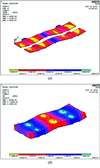Figure 1 Finite-element model of a deformable mirror with the fourth actuator applied at −500 V, the ninth actuator applied at 500 V, and the 15th actuator applied at −500 V. (a) The displacement in the vertical direction for the entire mirror. (b) The sum of the displacements for the piezoelectric materials.

The mirror shapes were measured by a Fizeau interferometer (Zygo, Verifire, 6″). It was necessary to wait for a sufficient time to allow the measured surface to reach a stable state at laser radiation. At each measurement, it was also necessary to wait for several minutes for the piezoelectric materials to become stable. Each measurement data point was the average result of ten measurements. The measurements of the piezoresponse function (PRF) were taken every time a new electrode was activated (Vannoni et al., 2016). The mirror figure error used for the PRF calculation was the difference between the mirror shapes obtained before and after this activation.

The in situ speckle scanning metrology measurements were performed at the BL15U1 beamline of the SSRF. The X-ray energy was 10 keV. The setup of the measurement is illustrated in Fig. 2. The deformable mirror was mounted with the vertical deflection on the sample manipulator of l1 = 1280 mm upstream of the sandpaper. The grazing-incidence angle for our experiment was at the total reflection θ = 0.213°. The average pore size of the sandpaper was 2.0 µm. The detection system, which was placed at l2 = 270 mm behind the mirror, was a microscope objective lens system (Optique Peter) with a magnification of 10 coupled to a CMOS camera (Hamamatsu). The effective pixel size was p = 0.65 µm. The exposure time was 100 ms. During the scanning measurements, the sandpaper was driven at a nano-precision motion stage (PI) with a step size of μ = 0.25 µm along the vertical direction Z, and 101 speckle patterns were recorded during each scan. Photographs of the deformable mirror, sandpaper and detector systems are shown in Fig. 3.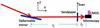Figure 2 Sketch of the in situ speckle scanning measurement setup.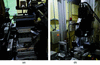Figure 3 Photographs of (a) the deformable mirror and (b) the sandpaper and detector system.

### 3. Method of figure correction

For a reflective mirror, since the slope is small compared with unity, the curvature C is approximately equal to the first derivative of the slope s and the second derivative of the figure height h. The phase errors are directly related to the figure errors Δh by the formula ΔΦ = 2kΔhsinθ, where k is the wavenumber and θ is the grazing-incidence angle. The phase errors determine whether the focusing satisfies the Rayleigh criterion in a coherent condition.

Huang et al. (2016) presented two methods for controlling the deformable mirror during inspection: a windowed Fourier ridge and weighted polynomial fitting. These methods regarded each actuator influence as a windowed function, either Gaussian or polynomial, to segment fit a curve of the slope error. These methods proved to be very effective for a 700 mm-long mirror. However, for a shorter mirror such as a 100 mm-long mirror with 18 actuators, these methods have their limitations. When the layout of the actuators is very compact, the influence of each actuator covers several adjacent actuators. In this case, the PRFs of the actuators were significantly overlapped. Using a windowed function to evaluate the slope error of an actuator section was not accurate due to these overlapped areas. This slows down the speed of convergence as well. To approach a real mirror shape, a global algorithm fitting based on the consideration of all actuators should have been more effective. A second consideration was calculating the curvature with the derivative of the slope error, or even the second derivative of the figure error would produce serious noise for real experimental data. This process had to introduce data smoothing or polynomial fitting, which made the automatic adaptive process complicated and uncontrolled. The figure error calculated from the integration process of the curvature or the slope could not be influenced by experimental noise, and it was more sensitive than fitting the curvature or the slope error. In this paper, we developed a global fitting method, the particle swarm optimization algorithm (Kennedy & Eberhart, 1995), to fit the figure error directly and improve the sensitivity and avoid introducing noise.

If the piezoresponse coefficient of the ith piezo (the area deposited the ith actuator) is defined as Ki, the measured piezoresponse coefficient is Ki′ = Ki + ΔKi. ΔKi is the experimental error of the ith piezo. The target mirror tangential curvature on the ith actuator is Cig. Initially, the input voltage Ui,0 = Cig/Ki′. Then the measured tangential curvature on the ith actuator is Ci,1m = KiUi,0 = (1 − ΔKi/Ki′)Cig. Based on the relationship ΔU = ΔC/K′, the ith voltage is upgraded to be Ui,1 = Ui,0 + ΔUi,1 = (1 + ΔKi/Ki′)Cig/Ki′. Then the new measured tangential curvature is Ci,2m = KiUi,1 = [1 − (ΔKi/Ki′)2]Cig. Based on the jth iterative raising of the voltages Ui,j = Ui,j–1 + ΔUi,j, the measured tangential curvature and the applied voltages are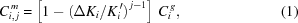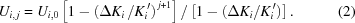The tangential curvatures approach the target values rapidly in exponential form. Accurate measured piezoresponse coefficients based on the PRF can decrease the iteration number. If the measurement error is 10%, only three iterations can reduce the error to 0.1%. If the error is 90%, 65 iterations are needed to reach this level. Each PRF can be assumed to be a Gaussian function gi,j(x) with a measured center position Xc, an amplitude A that is proportional to the curvature C, and a full width at half-maximum (FWHM). The merit function in the jth iteration can be expressed as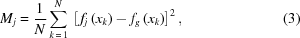where N is the sampling number, fg(xk) is the target mirror figure shape and fj(xk) is the figure shape calculated by fj(xk) =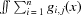. Fig. 4shows a flowchart of the entire iterative procedure used to approach a target mirror shape.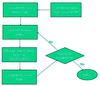Figure 4 An iterative process to approach the target mirror shape.

### 4. Piezoresponse

To determine the optimum voltages to control the mirror shape for a target figure, the PRFs of the deformable mirror were measured using the Zygo Fizeau interferometer, as shown in Fig. 5. In the same figure, the ANSYS simulations of two types of deformable mirror are compared. Fig. 6shows the center positions and the FWHMs of the measured PRFs and the simulated PRFs of the two types of deformable mirrors. The results show that the simulated curvatures of Type 1 were more than five times larger than the simulated curvatures of the segmented PZT (Type 2). The measured results were slightly smaller than the simulated ones. The center positions of the measured PRFs agreed with the simulated PRFs except for the actuators near both ends. It is noteworthy that the PRFs near the ends had significant small amplitudes and offset positions. These would influence the capability of deformation near the ends. The measured PRFs had wider response ranges, covering the areas of about four more adjacent actuators than the simulated PRFs. The measured FWHMs were close to those of Type 2. We also simulated the clamping simulations. The simulation results showed that the clamping forces of the springs at both the sides and the ends had weak influences on the mirror tangential shape even if providing rigid clamping.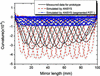Figure 5 Measured piezoresponse function (curvature per volt) of the prototype compared with the simulation results based on the two types of continuous and segmented PZT layers.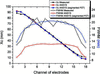Figure 6 Comparison of the center positions and the FWHMs of the measured PRFs and the simulated PRFs of the two types of deformable mirrors.

The prototype needed several minutes to stabilize. The PZT layer showed good short-term stability (<0.5%) and repeatability (<0.5%), but it was capable of deviating after a relatively long period of time such as several hours (∼1.5% h−1). In a similar manner to previous studies, Fig. 7exhibits good linearity of curvature in one charging or discharging process, but a hysteresis effect was found. This hysteresis effect mainly occurred because the strain of the PZT material could not return to the same state after charging or discharging.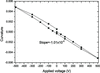Figure 7 Hysteresis measurements obtained by the charging or discharging process of the voltage range from −500 to 500 V.

### 5. Ex situ approach to a given figure

A target mirror figure was given, as shown in Fig. 8(a). The maximum deformation was 350 nm. The iterative method based on the description in Section 3was used for the real piezoelectric deformable mirror approach to this figure. The initial applied voltage was based on the measurement of the PRFs. Since every figure might correspond to the multiple optimization solution of voltages, after the first optimization the latter optimization process will be limited in a small search range of voltages such as 50 V to avoid large voltage changes of the actuators. This may result in an instability of the mirror figure. The results in Fig. 8(b)show that the RMS difference for the first measurement from the target figure was 44 nm. After 14 iterations this value decreased to 7 nm, which was obviously smaller than the figure error of the mirror itself. The main difference occurred at both ends of the mirror since the deformable capabilities of the actuators near the ends were relatively weak, and the curvatures were difficult to estimate accurately. If the data near the edges were not considered, the RMS difference of the figure error would be only 4 nm, and the RMS slope error would be only 0.5 µrad. Based on the estimation in equation (1), the RMS ΔKi/Ki was ∼80%. Because most of the errors were from the actuators near both ends, ΔKi/Ki near the center was smaller than 30%, whereas ΔKi/Ki near both ends was more than doubled, so the optimization could not be convergent. Fig. 9shows the voltage changes during the global optimization at each iteration.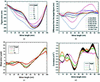Figure 8 The iterative process of the mirror figure approach. Comparison of the (a) figure errors, (b) differences of the figure errors from the target figure, (c) slope errors and (d) curvatures.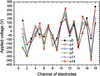Figure 9 Voltages suggested by global optimization at each iteration.

### 6. In situ wavefront correction

When a deformable mirror is mounted on a real beamline, it should achieve wavefront compensation and correction. The target slope error was zero. The in situ wavefront measurement was based on the X-ray speckle scanning technique. This technique has proven to be very effective for in situ determination of the figure errors of a bimorph mirror (Wang et al., 2015a,b). By scanning the sandpaper, a stack of speckle patterns at equidistant positions to the sandpaper can be obtained. The intensity profiles of all ith and jth rows in these patterns can be picked up and used to build two new images; i.e. two rows of data (ith and jth) can be expanded into two images with the width of the pattern number. By using the digital image correlation method (Pan et al., 2009), the shift of the maximum correlation coefficient i,j = argmax(Ii*Ij) between two images revealed the local wavefront distortion. The * symbol represents the correlation operator. The local wavefront curvature can be expressed as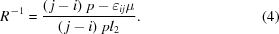Fig. 10presents the local wavefront curvatures in the detection plane. The results reveal that the mirror had a slightly convex surface rather than an ideal plane. Using the iterative method described in the last section, after only three iterations the local wavefront curvatures approached flatness. By transferring the local wavefront curvatures to the mirror surface by a geometric relationship, the equivalent figure errors of the deformable mirror could be presented as shown in Fig. 11. After three iterations, the RMS and PV figure errors of the mirror were reduced from 7.1 to 0.7 nm and from 33.0 to 2.7 nm, respectively. The PV figure error was very close to the requirement of a future nanoprobe beamline. The total time for each iteration, including the metrology and compensation, was less than 3 min. Hence, this research proves the feasibility of fast wavefront measurement and compensation.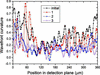Figure 10 Comparison of the local wavefront curvature in the detection plane after the phase compensations.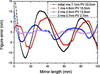Figure 11 After three iterations, the equivalent figure errors of the mirror showed an obvious improvement.

### 7. Conclusion

In this study, we made a prototype phase compensator based on Osaka's monomorphic design. An FEA model was developed to simulate the different actuator distribution and clamping schemes. We found that the types with actuators on the continuous PZT layers had a significantly larger piezoresponse than those on the segment PZT layers. The clamping had no obvious influence on the mirror figure based on the simulations. The piezoelectric deformable mirror had good short-time stability and repeatability after applied voltages, and it had ∼1.5% deviation per hour for long-time use. The hysteresis effect was found in one charging and discharging process. The main goal for this research was to develop an iterative global optimization method to approach a target figure height or figure slope. Strict recurrence formulas of the voltages were deduced, and a suitable merit function based on the target figure height or slope was used to carry out the optimization process. Different from the previous method based on the local curvature of a single actuator, a global PSO algorithm was used to minimize the merit function in each iteration to find optimal voltage distribution more quickly. Using more than ex situ Fizeau interferometry or in situ speckle scanning metrology, the iterative global optimization method helped the accurate metrology approach a target figure or an ideal wavefront in several iterations. This research can be significantly useful for future quick wavefront correction in a nanoprobe system.

### Acknowledgements

The authors would like to thank their colleague Dr Hongxin Luo's kindly help for the Fizeau interferometry measurement. They also thank their colleagues Dr Fen Tao and Dr Haipeng Zhang's help for early exploration of the experiments at the imaging beamline BL13W of SSRF. We thank LetPub (https://www.letpub.com) for its linguistic assistance during the preparation of this manuscript.

### Funding information

The following funding is acknowledged: National Natural Science Foundation of China (grant Nos. 11775295, U1432244); Youth Innovation Promotion Association.

### ReferencesJOURNAL OFSYNCHROTRONRADIATION